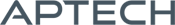# CurveFit

CurveFit

Availability: Out of stock

Given data and a procedure for computing the function, CurveFit will find a best fit of the data to the function in the least squares sense.
Overview

## CurveFit

Given data and a procedure for computing the function, CurveFit will find a best fit of the data to the function in the least squares sense.

Platform: Windows, Mac, and Linux.

Requirements: GAUSS/GAUSS Light v8.0 or higher.

Features

### Special Features

• Weight observations
• Multiple dependent variables
• Bootstrap estimation
• Histogram and surface plots of bootstrapped coefficients
• Profile t, and profile likelihood trace plots
• Levenberg-Marquardt descent method
• Polak-Ribiere conjugate gradient descent method
• Ability to activate and inactivate coefficients
• Heteroskedastic-consistent covariance matrix of coefficients

### Bootstrap Estimation

CurveFit includes special procedures for computing bootstrapped estimates. One procedure produces a mean vector and covariance matrix of the bootstrapped coefficients. Another generates histogram plots of the distribution of the coefficients and surface plots of the parameters in pairs. The plots are especially valuable for nonlinear models because the distributions of the coefficients may not be unimodal or symmetric.

### Profile t, and Profile Likelihood Trace Plots

Also included in the module is a procedure that generates profile t trace plots and profile likelihood trace plots using methods described in Bates and Watts, "Nonlinear Regression Analysis and its Applications". Ordinary statistical inference can be very misleading in nonlinear models. These plots are superior to usual methods in assessing the statistical significance of coefficients in nonlinear models.

### Descent Methods

The primary descent method for the single dependent variable is the classical Levenberg-Marquardt method. This method takes advantage of the structure of the nonlinear least squares problem, providing a robust and swift means for convergence to the minimum. If, however, the model contains a large number of coefficients to be estimated, this method can be burdensome because of the requirement for storing and computing the information matrix. For such models the Polak-Ribiere version of the conjugate gradient method is provided, which does not require the storage or computation of this matrix.

### Multiple Dependent Variables

CurveFit allows multiple dependent variables using a criterion function permitting the interpretation of the estimated coefficients as either maximum likelihood estimates or as Bayesian estimates with a noninformative prior. This feature is useful for estimating the parameters of "compartment" models, i.e., models arising from linear first order differential equations.

Product Inquiry

8 + 4 = enter the result ex:(3 + 2 = 5)

﻿